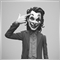# Low since last High149

Hello,

I 'm looking at an expert based on the High over last 10 Bars (H1)

I want to calculate the Low since last High was touched

Here's what I did but the problem is it gives me the Low since a time equal to the beginning of the Bar where the High was hit and what I want is the exact time when the High was hit.

Thanks for looking,

```  bin = iHighest(Symbol(),Period(),MODE_HIGH,10,0) ;
Maximum = iHigh(Symbol(),Period(),bin);
rbin = iLowest(Symbol(),Period(),MODE_LOW,bin,0);
LowSinceMax = iLow(Symbol(),Period(),rbin);```20233

marsupilami: what I want is the exact time when the High was hit.
You can do it by watching price and remembering the time of each new high, but it can't recovered on start. The best you can do is look at the M1 chart for the bar in question.
On MT4: Unless the current chart is that specific pair/TF referenced, you must handle 4066/4073 errors.149

Thanks,

Something like that I guess (good only if Period() = 60 )

```    Maximum = iHigh(Symbol(),Period(),iHighest(Symbol(),Period(),MODE_HIGH,10,0));
bin = iHighest(Symbol(),1,MODE_HIGH,10*Period() + TimeMinute(iTime(Symbol(),1,0)),1) ;
rbin = iLowest(Symbol(),1,MODE_LOW,bin,0);
LowSinceMax = iLow(Symbol(),1,rbin ;

```16538

marsupilami:

Thanks,

Something like that I guess (good only if Period() = 60 )

as whroeder1 already mention, use M1 chart

```int bar = 3600 * 10; // last 10 hours

int shiftHighest  = iHighest(_Symbol,PERIOD_M1,MODE_HIGH,bar);
int shiftLowest   = iLowest(_Symbol,PERIOD_M1,MODE_LOW,bar);
double high = iHigh(_Symbol,PERIOD_M1,shiftHighest);
double low = iLow(_Symbol,PERIOD_M1,shiftLowest);
datetime hightime = iTime(_Symbol,PERIOD_M1,shiftHighest);
datetime lowtime = iTime(_Symbol,PERIOD_M1,shiftLowest);```149

```double shiftLowestsinceHigh = iLowest(Symbol, PERIOD_M1,MODE_LOW,shiftHighest);